## The Real Value of the `Repeat` Block

It is, of course, much easier to create this script...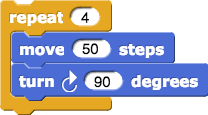...than to create this one: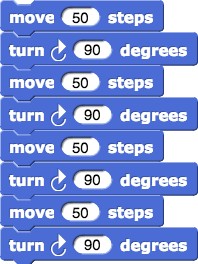Even more importantly, using `repeat` makes the structure of the program clearer. This is essential to good coding. Clearly structured code is easier to understand and easier to debug (to catch and fix errors). In the longer script above (without `repeat`), we'd have to count the parts to see if it's right. And it would be very easy to make a mistake if we were drawing a 20-sided figure instead of a 4-sided one!

1. Analyze structure: Here are four pictures.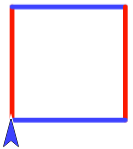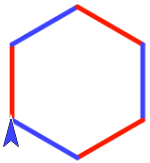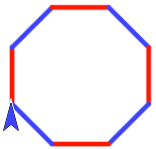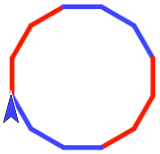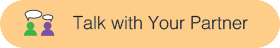Which one(s) of these pictures could be drawn by running the following script?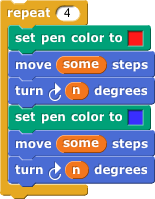2. For each picture, write a script similar to the one above that describes how to draw it. (You don't need to say how long each side is (some) or how many degrees to turn (n), but you do need to show what code is repeated and how many times it repeats.)
3. Experiment: Run yout scripts in snap and experiment with the number of degrees to make your pictures match the ones here. (Don't worry about matching the size exactly.)

1. Look for patterns.
Use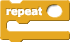to draw the following regular polygons using the smallest number of lines:
A regular polygon is a polygon in which all the sides are the same length and all the turning angles are the same. A square is a regular four-sided polygon.
• Equilateral Triangle
• Pentagon
• Hexagon
• Octagon

### The `Repeat` Block Self Check

Through how many degrees did your sprite turn altogether when you drew a pentagon?

72
108
360
540

What's the smallest number you can put into the blank numeric input of the `repeat` block below to produce this picture?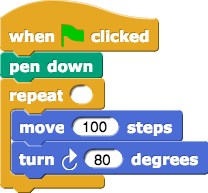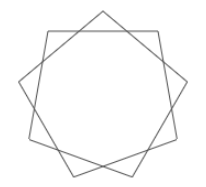9
5
10

1. Determine the turning angles to draw a 12-sided or 36-sided regular polygon.
2. Make small enough sides so that the shapes can fit on the stage. With more and more sides, the polygons get closer and closer to a circle. Is there a point at which the result is a circle?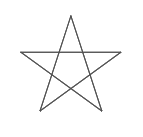3. The code in self check question 2 above created a regular 9-pointed star. Through how many degrees did the sprite turn altogether to draw that star?
4. Adjust the numbers in that script to get a regular 5-pointed star.
5. Through how many degrees did your sprite turn altogether when you drew the five-pointed star?
6. Find a starting direction so that the star sits "straight."